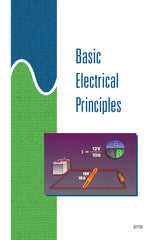#### Get the Study Guide for this videoFollow the video session-by-session with this handy book. Color illustrations throughout. \$15

Instructor Guide  available

# Basic Electrical Principles - Instant Video

##### Summary

This 24-minute video delivers the foundation for a basic understanding of electrical theory and circuit analysis. The video begins with a review of Ohm's law and Kirchhoff's current and voltage laws, and progresses to an explanation of power and energy in DC circuits. Shows in simplified form, how power and energy are metered.

##### Training Sessions

Ohm's Law – 5 minutes
Identifies the three basic parts of a simple electrical circuit and the electrical property associated with each. Using Ohm's law, describes the relationship between current, voltage, and resistance in a circuit. Demonstrates how to calculate the current, voltage, or resistance of a simple circuit, when the other two quantities are known
.

Series Circuits – 6 minutes
Identifies the characteristics of a series circuit. Explains the principles of series circuits relating to current, total resistance, and Kirchhoff's voltage law.

Parallel Circuits – 7 minutes
Identifies the characteristics of a parallel circuit. Explains the principles of parallel circuits relating to voltage, branch currents, Kirchhoff's current law, and total resistance.

Power and Energy – 6 minutes
Defines electric power. Explains how power, current, or voltage can be calculated for a DC circuit when the other two quantities are known. Defines energy and explains how energy is calculated.

Item Code: BEP-IV